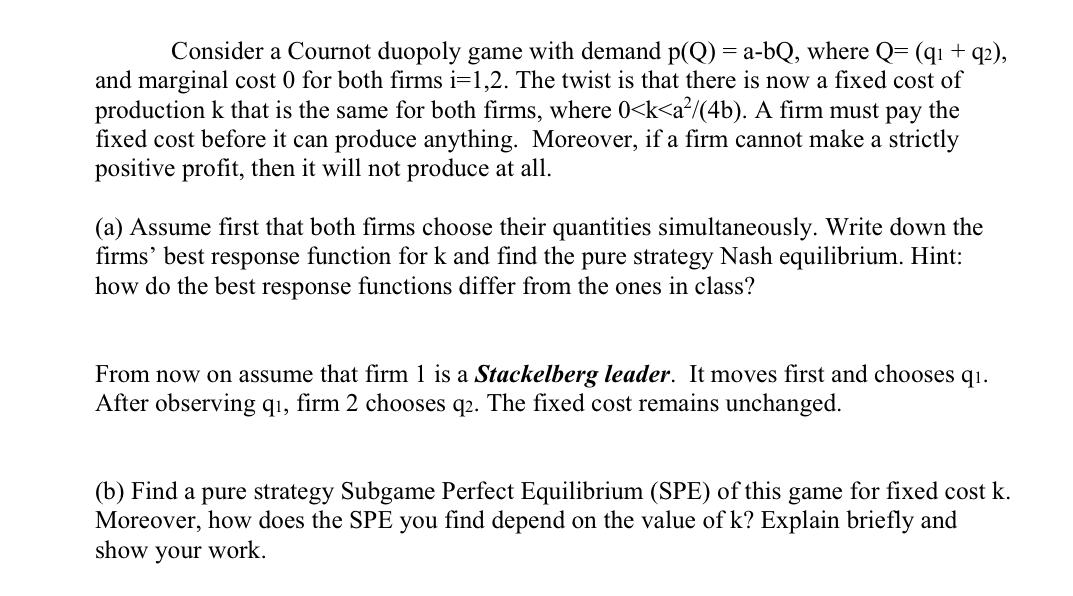### Create an Account

Already have account?

### Forgot Your Password ?

Home / Questions / Consider a Cournot duopoly game with demand p(Q) = a-bQ, where Q=(qı + q2), and marginal c...

# Consider a Cournot duopoly game with demand p(Q) = a-bQ, where Q=(qı + q2), and marginal cost 0 for both firms i=1,2. The twist is that there is now a fixed cost of production k that is the same for b

Consider a Cournot duopoly game with demand p(Q) = a-bQ, where Q=(qı + q2), and marginal cost 0 for both firms i=1,2. The twist is that there is now a fixed cost of production k that is the same for both firms,Jun 10 2021 View more View Less

#### Answer (Solved)Subscribe To Get Solution